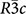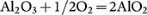## Introduction

Aluminum and oxygen are among the most abundant elements in the universe. Their only stable compound, alumina Al2O3, is widely used due to its mechanical properties (e.g. as an abrasive material) and due to its very wide band gap (for example, as an optical window material in shock-wave experiments).

Al2O3 in the corundum structure (space group) is an important mineral in the Earth's crust. Alumina is easily incorporated into many silicates and significantly affects their physical properties1,2. Several phase transitions have been theoretically predicted and experimentally confirmed to occur under pressure. It was shown that corundum transforms to the Rh2O3(II)-type structure (space group Pbcn) at ~80 GPa3,4 and to the CaIrO3-type phase (“post-perovskite”, space group Cmcm) above 130 GPa5,6. In 2007, Umemoto et al.7 predicted a further phase transition to a U2S3-type polymorph (space group Pnma) at ~370 GPa.

Given the high degree of ionicity of the Al-O bond (due to the large electronegativity difference, 1.8 on the Pauling scale) and the only possible oxidation states being +3 and −2 for Al and O, respectively, the only possible stable compound seems to be Al2O3. Of course, one can also imagine a peroxide with composition Al2(O2)3 = AlO3, but such a compound has never been reported.

While no other stable oxides are known, there is evidence for metastable AlO2, which was shown to form by an interfacial reaction in the presence of a kinetic constraint during diffusion-bonding of Pt and α-Al2O3. Raman spectroscopy has provided strong evidence for the presence of AlO28, which formed after heating for 24 hours in the temperature range 1200–1400°C. AlO2 is a “peroxide oxide”, i.e. contains peroxide (O22−) and oxide (O2−) ions. It was not clear whether AlO2 or other unusual oxides are stable at any pressure-temperature conditions.

Very recently, it has been shown that even in seemingly extremely simple systems, such as Na-Cl, totally unexpected compounds (Na3Cl, Na2Cl, Na3Cl2, NaCl3 and NaCl7) become stable under pressure – these have been predicted using evolutionary crystal structure prediction method USPEX and verified by experiments9. If such unusual compounds exist in the “trivial” Na-Cl system, one can expect similarly unusual compounds in nearly any other system under pressure. Here we test this hypothesis on the Al-O system and indeed predict that Al4O7 and AlO2 become thermodynamically stable under high pressure.

## Computational Methodology

To predict stable Al-O oxides and their structures, we used the evolutionary algorithm USPEX10,11,12 in its variable-composition mode13 at pressures 0, 50, 100, 150, 200, 300, 400, 500 GPa. The reliability of USPEX has been demonstrated many times before – e.g. Ref. 9, 14–18. Modern methods have shown remarkable power to predict novel unexpected compounds – e.g. in the Na-Cl9, Mn-B19, Mg-C20 and Na-Si21 systems. Stable compositions were determined using the convex hull construction: a compound is thermodynamically stable when its enthalpy of formation from the elements and from any other compounds is negative. Enthalpy calculations and structure relaxations were done using density functional theory (DFT) within the Perdew-Burke-Ernzerhof (PBE) generalized gradient approximation (GGA)22, as implemented in the VASP code23. These calculations were based on the all-electron projector-augmented wave (PAW) method24 and plane wave basis sets with the kinetic energy cutoff of 600 eV and uniform Γ-centered k-point meshes with reciprocal-space resolution of 2π*0.02 Å−1. The first generation of structures/compositions was produced randomly with the use of space group symmetries (using algorithm12); the lowest-fitness 60% of the structures/compositions were allowed to produce child structures/compositions (fitness being defined as the difference between enthalpy of the structure and the convex hull). Initial structures were allowed to have up to 20 atoms in the unit cell, but this range was allowed to change in subsequent generations as a result of evolution. Child structures/compositions were created in the following manner: 20% by random symmetric generator, 40% by heredity, 20% by softmutation and 20% by atomic transmutation. In this work, we first performed searches in the entire Al-O system with up to 20 atoms/cell and have found only Al2O3 and oxygen-enriched phases Al4O7 and AlO2. Then we did additional focused searches in a narrower compositional range Al2O3-O and obtained the same result.

After USPEX predictions, we selected structures on the convex hull and close to it and relaxed them carefully at pressures 0, 10, …, 520 GPa. These calculations have confirmed stability of three oxides – well-known Al2O3 and non-classical AlO2 and Al4O7. For these compounds, we also computed their electronic band structures. For accurate estimates of the band gaps, we have used the HSE hybrid functional25. Phonon frequencies throughout the Brillouin zone were calculated using the finite displacement approach as implemented in the Phonopy code26,27 and these calculations confirmed that these phases are dynamically stable at pressure ranges where our enthalpy calculations predict their thermodynamic stability.

## Results

### Stable compounds in the Al-O system

At all pressures in the range 0–500 GPa, the known compound - Al2O3 - is found to be thermodynamically stable. In agreement with previous works we find the same sequence of phase transitions – from corundum to the Rh2O3(II)-type structure at 100 GPa, then to the CaIrO3-type structure at 130 GPa and then to the U2S3-type phase at 394 GPa.

The computed thermodynamics of Al-O compounds are shown in Fig. 1. Al4O7 and AlO2 begin to show competitive enthalpies of formation at pressures above 300 GPa and have stability fields at 330–443 GPa and at >332 GPa, respectively. From Fig. 1, one can see that at 500 GPa the enthalpy of formation of AlO2 from Al2O3 and O is impressively negative, −0.12 eV/atom. The predicted pressure-composition phase diagram is shown in Fig. 2. To assess the effect of temperature, we performed quasi-harmonic free energy calculations for AlO2, Al2O3 and O. We found that temperature and zero-point vibrations stabilize AlO2 and expand its stability field: at T = 300 K it becomes stable at 321 GPa, at T = 2060 K at 300 GPa, at T = 3200 K at 280 GPa.

### Structures of stable compounds: Al4O7 and AlO2

Structures of the stable phases of Al2O3 have been discussed elsewhere, so here we focus only on the new compounds, Al4O7 and AlO2. Each of these compounds has only one stable structure up to 500 GPa and both contain at the same time oxide O2− and peroxide [O-O]2− anions, i.e. both can be described as “oxide peroxides”. At normal conditions, the O-O bond lengths are28 1.21 Å in the O2 molecule, 1.28 Å in the superoxide O2− ion and 1.47 Å in the peroxide O22− ion. In Al4O7 the O-O bond length is 1.43 Å at 400 GPa, in AlO2 it is 1.38 Å at 500 GPa – clearly indicating the presence of peroxide-ions.

The chemical formulas of these compounds can be obtained from Al2O3 by consecutive replacement of O2− by O22− (which has the same charge): taking two formula units Al4O6 and replacing O2→O22−, we obtain Al4O5(O2) = Al4O7 and doing the same replacement again, we obtain Al4O4(O2)2 = AlO2. These are indeed the structural formulas: Al4O5(O2) for Al4O7 and Al4O4(O2)2 for AlO2. These structures are shown in Figs. 3 and 4 and their parameters are given in Table 1.

## Discussion

### Properties of the new phases

Phonon dispersion curves of Al4O7 and AlO2, computed at 400 and 500 GPa, respectively, are shown in Fig. 5. Both phases are dynamically stable and display a continuum of phonon energies, i.e. absence of decoupled O-O vibrational modes of peroxo-groups, because at high pressure Al-O modes have frequencies comparable to O-O modes. At the same time, in the electronic structure, there are clearly defined dispersive bands of peroxo-groups and these play an important role, as we discuss below. Both phases are dynamically and mechanically stable, as shown by their computed phonons, elastic constants and evolutionary metadynamics29 simulations, also enabled in the USPEX code and allowing one to explore possible phase transitions. We have confirmed that there are indeed no distortions or modulations that could lead to more stable structures.

All the predicted phases are insulating and show very distinct electronic structure compared with Al2O3. At 400 GPa, the computed DFT band gaps are 6.93 eV for Pnma-Al2O3, 2.51 eV for Al4O7, 2.92 eV for AlO2. We recall that DFT calculations significantly underestimate band gaps, while hybrid functionals and GW approximation give much better band gaps, typically within 5-10% of the true values. Fig. 6 shows band gaps as a function of pressure, computed using the GGA (PBE functional), hybrid HSE functional25 and GW approximation30,31; one can see that GGA band gaps are ~30% underestimates; HSE band gaps practically coincide with the most accurate GW values for AlO2, but are 0.2–1.1 eV lower for Al2O3 and Al4O7. At all levels of theory, Al4O7 and AlO2 come out to have band gaps ~2 times lower than the band gap of Al2O3.

This band gap reduction for Al4O7 and AlO2 originates from the additional low conduction band in the middle of the band gap. Our calculations (Fig. 7) show that these low conduction bands can be unequivocally assigned to the peroxo-groups. In both Al4O7 and AlO2, both gap states - the HOMO (highest occupied molecular orbital) and LUMO (lowest unoccupied molecular orbital) - come from peroxo-groups. Together with low compressibility of the peroxo-groups (between 300 GPa and 500 GPa, the O-O distance changes from 1.37 to 1.38 Å and from 1.46 to 1.42 Å in AlO2 and Al4O7, respectively), this explains why the band gaps of Al4O7 and especially AlO2 are practically independent of pressure in a wide pressure range (Fig. 6). As Fig. 8 shows, projected densities of states show only small contributions from Al, thus indicating a high degree of ionicity. Indeed, Bader charges32 are +2.44 of Al, −0.83 of O1 (peroxide anion) and −1.61 of O2 (oxide anion) in AlO2 at 400 GPa.

While the band gaps computed by DFT (PBE functional) are, as expected, significantly underestimated, the energetics are accurate. We have tested this by computing the energy and enthalpy of the reactionusing the combined exact exchange (EXX) and random phase approximation (RPA) technique33,34,35. At 300 GPa we obtained the following energies (enthalpies) for this reaction: 0.1727 eV/atom (−0.0113 eV/atom) for the RPA+EXX method and 0.1782 eV/atom (0.0330 eV/atom) for PBE. At 500 GPa the results are 0.1284 eV (−0.1200 eV/atom) for RPA+EXX and 0.1371 eV (−0.1150 eV/atom) for PBE. These calculations fully confirm our findings and give additional insight:

1. 1

In both PBE and EXX+RPA the new compounds are stabilized by the P*V-term in the free energy, rather than by the internal energy. This originates from the low packing efficiency in elemental oxygen, which remains a molecular solid in the entire pressure range studied here. For this reason we can expect increased reactivity of oxygen and stabilization of oxygen-rich compounds (such as peroxides) at high pressures.

2. 2

Results of the PBE and EXX+RPA are quantitatively similar, especially at 500 GPa, where the difference is only 5 meV/atom.

3. 3

At the EXX+RPA level of theory the new compounds predicted here are even more stable than at the PBE level.

## Conclusions

Systematic search for stable compounds in the Al-O system at pressures up to 500 GPa revealed two new stable compounds (AlO2 and Al4O7); their stability fields are above 332 GPa and in the range 330–443 GPa, respectively. Our analysis reveals that insulating compounds AlO2 and Al4O7 exhibit significantly ionic character, both contain peroxide [O-O]2− and oxide O2− anions and therefore belong to the exotic class of “peroxide oxides”. Electronic levels of the peroxo-groups form gap states (“low conduction band”) that lead to a twofold lowering of the band gap relative to Al2O3. Our preliminary results show that the formation of peroxo-ions and stabilization of peroxides under pressure occur in many oxide systems and this phenomenon may play an important role in planetary interiors, with their high pressures and abundance of oxygen atoms.